• matlab中plot函数用法
万次阅读 多人点赞
2019-03-06 14:41:15

线条、颜色等参数：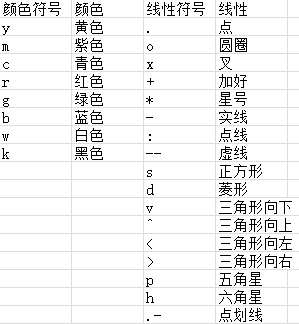1.简单的2维直线图 : plot(x,y)
同一坐标显示n条线：plot(x,y1,x,y2，…)

x = 0:pi/10:2*pi;
y = sin(x);
figure;
hold on;
plot(x,y)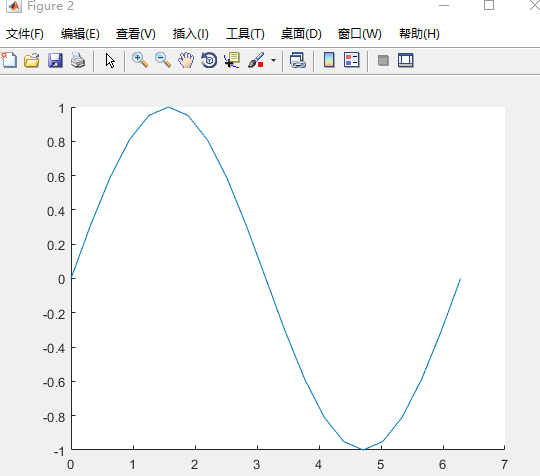2.plot(X):X是矩阵，表示矩阵的每一行都画一条线，将显示n条线。

X=rand(3,3); %随机生成3*3矩阵
figure;
hold on;
plot(X)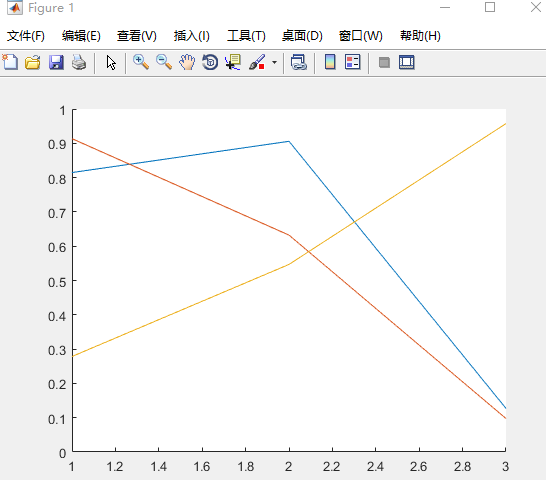3.画带形状的图

plot(X(pos, 1), X(pos, 2), 'k+','LineWidth', 2, 'MarkerSize', 7);
plot(X(neg, 1), X(neg, 2), 'ko', 'MarkerFaceColor', 'y','MarkerSize', 7);

X(pos, 1), X(pos, 2)表示横纵坐标
'k+'表示线性为加号型、黑色
LineWidth', 2表示线宽为2
'MarkerSize', 7表示形状大小为7
'MarkerFaceColor', 'y'表示形状填充颜色为黄色
另：MarkerEdgeColor','b'表示方框边缘颜色为蓝色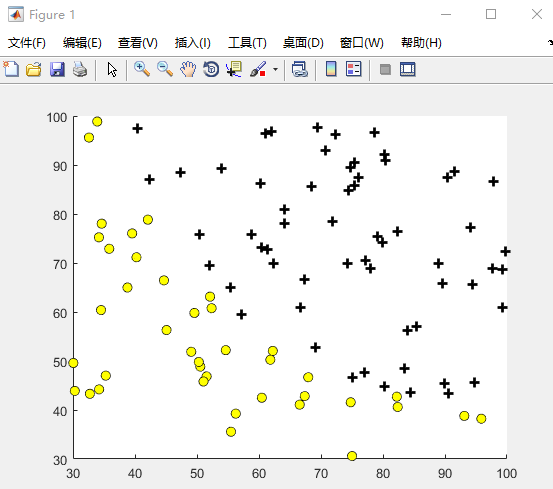matlab
更多相关内容
• ## MATLAB中plot函数的用法

万次阅读 多人点赞 2018-04-23 15:47:04
使用plot绘制二维图像本文转自http://blog.sina.com.cn/s/blog_d8f783c90102woqb.html以及https://blog.csdn.net/alvern_zhang/article/details/51153058MATLAB中plot函数常常被用于绘制各种二维图像，其用法也是...

## 使用plot绘制二维图像

本文转自http://blog.sina.com.cn/s/blog_d8f783c90102woqb.html

以及https://blog.csdn.net/alvern_zhang/article/details/51153058

MATLABplot函数常常被用于绘制各种二维图像，用法也是多种多样，本文仅介绍plot函数的基本用法——使用plot函数绘制二维点图和线图。plot函数的一般调用形式如下：

plot(X, Y, LineSpec)

其中X由所有输入点坐标的x值组成，Y是由与X中包含的x对应的y所组成的向量。LineSpec是用户指定的绘图样式，主要选项如下：

 Specifier Line Style（线型） --- 实线（默认样式）虚线（短划线） : 点线 -. 点划线

 Specifier Marker（坐标点样式） o 圆 + 加号 * 星号 . 点 x 十字 s 正方形 d 菱形 ^ 上指向三角形 v 下指向三角形 > 右指向三角形 < 左指向三角形 p 五角星 h 六角形

 Specifier Color y 黄色 m 品红 c 蓝绿色 r 红色 g 绿色 b 蓝色 w 白色 k 黑色

例如：’--or’表示坐标点为圆圈标志，且线型为红色短划线的绘图样式。

### 注意

在同时绘制多条曲线时，如果没有指定曲线属性，plot按顺序循环使用当前坐标系中ColorOrder和LineStyleOrder两个属性。

默认情况，MATLAB在每次调用plot函数时将ColorOrder和LineStyleOrder自动重置为DefaultAxesColorOrder和DefaultAxesLineStyleOrder。Default**属性我们可以自定义，有效期至MATLAB关闭，Matlab下次启动时将Default**属性重置为厂家设置(Factory)

set(0,’DefaultAxesColorOrder’,’r|g|b|k’,…’DefaultAxesLineStyleOrder’,’-|-.|–|:’)

使用hold all命令可以阻止调用plot函数时自动重置ColorOrder和LineStyleOrder属性，而是循环使用。注意hold on只是使多次绘制的图形叠加（相当于NextPlot），但不能阻止属性重置。

另外我们可以通过下面四个属性设置标识符的颜色和大小

LineWidth——指定线宽

MarkerEdgeColor——指定标识符的边缘颜色

MarkerFaceColor——指定标识符填充颜色

MarkerSize——指定标识符的大小

注意上面四个属性是针对当前坐标系中所有曲线的

### 实例

X=1:10;
% 两个都是数组，必须具有相同的尺寸
X1=[X;X;X]’;%10×3
Y1=rand(10,3)+1;%10×3% 其中一个为向量，另一个为数组，自动匹配尺寸相等方向
X2=1:0.1:10;%1×91
Y2=[sin(X2);cos(X2)]’;%91×2% 其中一个是标量，另一为矢量，绘制垂直坐标轴的离散点
X3=1:10;
Y3=-0.5;
fh=figure(‘numbertitle’,’off’,’name’,’PLOT Usability Demo’);%创建figure对象
ah=axes;%创建axes对象
h=plot(…%返回所有曲线句柄
ah,…%指定坐标系，可以省略，此时默认gca
X1,Y1,…%坐标数据
‘-.^’,…%曲线属性，可以省略或部分省略，此时自动选择
X2,Y2,…
‘m-‘,…
X3,Y3,…
‘o’,…%注意此组数据设置线型和颜色无效，因为默认绘制离散点
‘LineWidth’,2,…%线宽
‘MarkerEdgeColor’,’k’,…%标识符边缘颜色
‘MarkerFaceColor’,’r’,…%标识符填充颜色
‘MarkerSize’,8)%标识符大小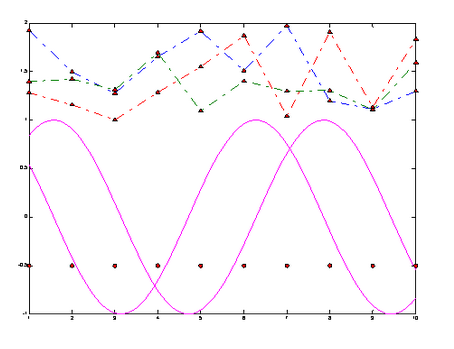举例如下：

定义x02pi之间的一组向量，且x向量中相邻两个值之间的增量为pi/100。定义y向量中的值为x向量的sine值。

x = 0:pi/100:2*pi;

y = sin(x);

figure 打开新的绘画窗口，可省略该句

plot(x,y) %采用默认样式，绘制实线

x = 0:pi/20:2*pi;

y = sin(x);

plot(x,y,'--or') %坐标点为圆圈标志，且线型为红色短划线的绘图样式

注：可以根据上述LineSpec表格自由组合线型样式。

在不关闭绘图窗口的前提下，还可以为图像添加标题，x轴和y轴标签，例如：

xlabel('x')

ylabel('sin(x)')

title('Plot of the Sine Function')

想要在现有的图片上添加另外一条线，可以使用hold语句，例如：

x = 0:pi/100:2*pi;

y = sin(x);

plot(x,y)

hold on

y2 = cos(x);

plot(x,y2,'r:')

legend('sin','cos') %为图片添加图例## 使用plot3绘制三维图像

MATLAB中有许多函数可以用来绘制三维图像，在此仅对plot3函数进行简要介绍，有兴趣的同学可以自行学习。plot3函数的调用形式如下：

plot3(X, Y, Z,LineSpec)

例如：

z=0:pi/50:10*pi;

x=sin(z);

y=cos(z);

plot3(x,y,z)

展开全文matlab
• 使用plot函数绘制图像 plot(x, y) 对图像属性进行设置，可以使用以下代码： p = plot(x, y); p.LineWidth = 2; %线宽为2 p.Color = 'r'; % 红色线条 p.Marker = '*' ; % 设置标记类型 p.MarkerSize = 6; %设置标记...

随机生成一组数据：

x = [1:1:10];
y = rand(1, 10);


使用plot函数绘制图像

plot(x, y)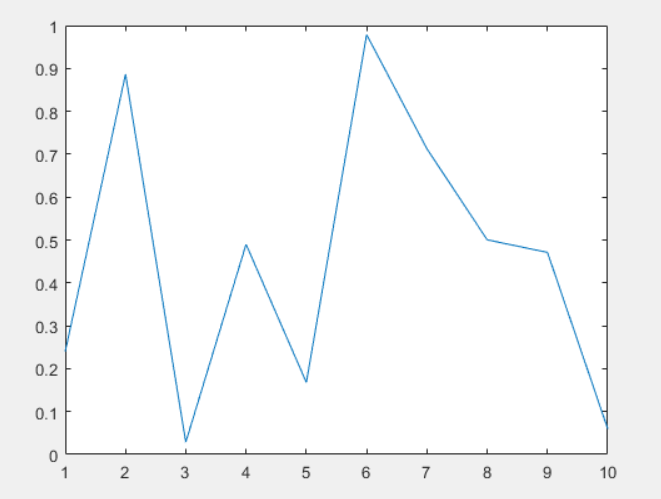对图像属性进行设置，可以使用以下代码：

p = plot(x, y);
p.LineWidth = 2; %线宽为2
p.Color = 'r';   % 红色线条
p.Marker = '*' ; % 设置标记类型
p.MarkerSize = 6; %设置标记大小
p.MarkerEdgeColor = 'b'; %设置标记边缘颜色
p.MarkerFaceColor = 'r'; %设置标记填充颜色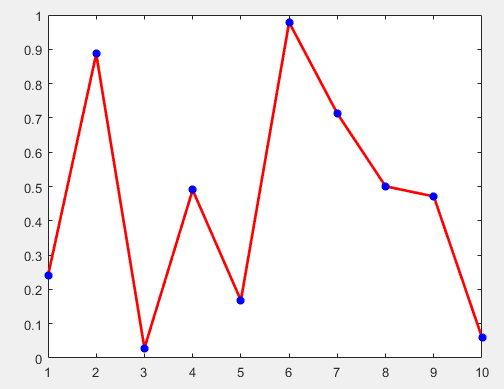set(gca, 'xtick', [3:1:12])  %自定义横坐标显示范围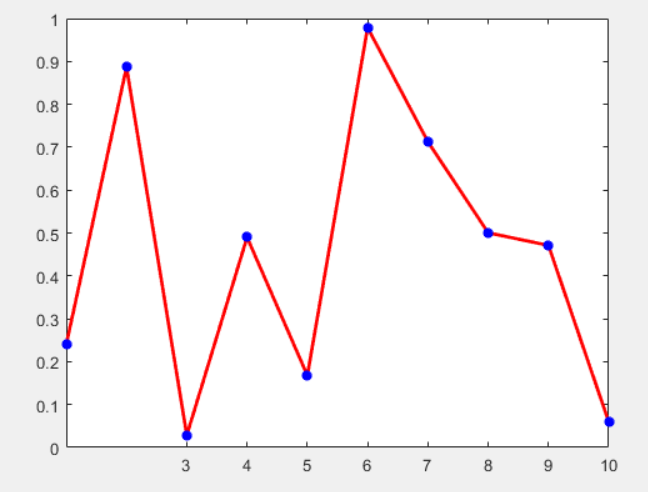set(gca, 'xticklabel', {'F1', 'F2', 'F3', 'F4', 'F5', 'F6', 'F7', 'F8', 'F9', 'F10' })  %自定义横坐标显示标签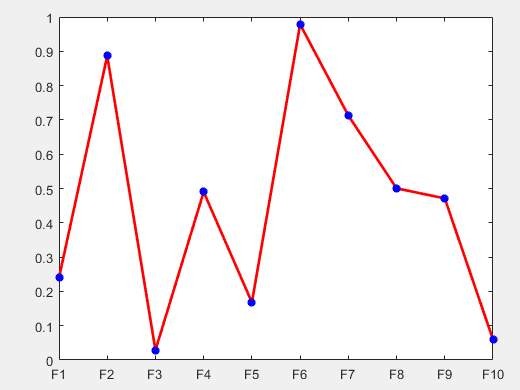添加图例

legend('Random Points')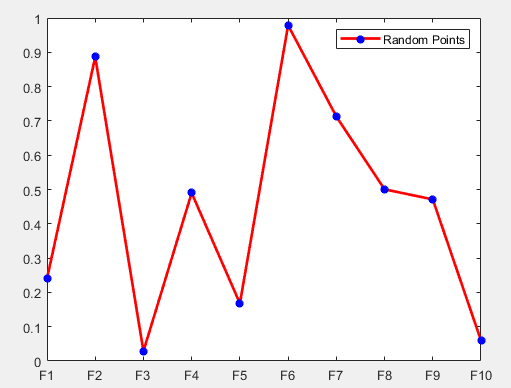设置坐标轴上下限：

axis([xmin,xmax,ymin,ymax]);


设置图片大小：

set(gcf,‘Position’,[x1,y1,dx,dy]);


x1和y1是图的左下角坐标， dx和dy是图沿着x和y方向的大小， 比如：

set(gcf,"Position",[347,162,380,300]);


图例的相关设置：
图例中字体及大小：

legend("FontName","Times New Roman","FontSize",7,LineWidth",1.5);


图例中各个量及位置：

legend("y","zc","location","SouthEast");


坐标轴的名称的相关设置：

x轴的名称及字体和大小：

xlabel("x(m)","FontName","Times New Roman","FontSize",7);


y轴的名称及字体和大小：

ylabel("zc(m) and y(m)","FontName","Times New Roman","FontSize",7,LineWidth",1.5);


坐标轴的字体及大小，以及坐标轴的刻度间隔

set(gca,'xtick', [1:1:10], "FontName","Times New Roman","FontSize",7,"LineWidth",1.5);


图名的字体及大小设置

title("yslice:600m;zslice:150m","FontName","Times New Roman","FontSize",7);


坐标轴反向

set(gca,"zdir","reverse"); %将坐标轴数值反向

展开全文matlab 开发语言
• ## MATLAB：plot函数详解

万次阅读 多人点赞 2021-08-08 20:50:29
本文介绍了MATLAB中plot函数用法，方便日后适用

## 1 plot 函数语法

plot：绘制二维线图

NO.1 绘制横轴为X，竖轴为Y二维线图，Y值与X值一一对应。

plot(X,Y)


如果 X 和 Y 都是向量，则它们的长度必须相同。plot 函数绘制 Y 对 X 的图。

如果 X 和 Y 均为矩阵，则它们的大小必须相同。plot 函数绘制 Y 的列对 X 的列的图。

如果 X 或 Y 中的一个是向量，而另一个是矩阵，则矩阵的各维中必须有一维与向量的长度相等。如果矩阵的行数等于向量长度，则 plot 函数绘制矩阵中的每一列对向量的图。如果矩阵的列数等于向量长度，则该函数绘制矩阵中的每一行对向量的图。如果矩阵为方阵，则该函数绘制每一列对向量的图。

如果 X 或 Y 一个为标量，而另一个为标量或向量，则 plot 函数会绘制离散点。但是，要查看这些点，必须指定标记符号，例如 plot(X,Y,‘o’)。

NO.2 设置线型、标记符号和颜色。

plot(X,Y,LineSpec)


LineSpec — 线型、标记和颜色

线型、标记和颜色，指定为包含符号的字符向量或字符串。符号可以按任意顺序显示。不需要同时指定所有三个特征（线型、标记和颜色）。例如，如果忽略线型，只指定标记，则绘图只显示标记，不显示线条。

示例： ‘–or’ 是带有圆形标记的红色虚线

线型说明
- (默认)实线
--虚线
:点线
-.点划线
标记说明
none（默认）不显示
o圆圈
+加号
*星号
.
x叉号
_水平线条
|垂直线条
s方形
d菱形
^上三角
v下三角
>右三角
<左三角
p五角形
h六角形
颜色说明
r红色
g绿色
b蓝色
w白色
k黑色
y黄色
m品红/洋红
c青蓝

NO.3 绘制横轴为X，竖轴为Y的多组二维线图，Y值与X值一一对应，所有线条都使用相同的坐标区。

plot(X1,Y1,...,Xn,Yn)


NO.4 设置每个线条的线型、标记符号和颜色。

plot(X1,Y1,LineSpec1,...,Xn,Yn,LineSpecn)


可以混用 X、Y、LineSpec 三元组和 X、Y 对组：例如

plot(X1,Y1,X2,Y2,LineSpec2,X3,Y3)


NO.5 创建 Y 中数据对每个值索引的二维线图。

plot(Y)


如果 Y 是向量，x 轴的刻度范围是从 1 至 length(Y)。

如果 Y 是矩阵，则 plot 函数绘制 Y 中各列对其行号的图。x 轴的刻度范围是从 1 到 Y 的行数。

如果 Y 是复数，则 plot 函数绘制 Y 的虚部对 Y 的实部的图，使得 plot(Y) 等效于 plot(real(Y),imag(Y))。

也可以设置线形、标记和颜色

plot(Y,LineSpec)


NO.6 使用一个或多个 Name,Value 对组参数指定线条属性。如线宽、线形、线条颜色等。

plot(___,Name,Value)


## 2 示例

### 2.1 plot(X,Y) | plot(X1,Y1,X2,Y2)

以绘制三角函数曲线为例

代码1：

clc;
clear;

% 绘制
x = linspace(0,2*pi);
y = sin(x);
plot(x,y)
title('y=sin(x)')
xlabel('X(m)')
ylabel('Y(m)')


结果展示：代码2：

clc;
clear;

% 绘制
x = linspace(0,2*pi);
y1 = sin(x);
y2 = cos(x);
plot(x,y1,x,y2)
title('y1=sin(x),  y2=cos(x)')
xlabel('X(m)')
ylabel('Y(m)')


结果展示：### 2.2 plot(X,Y.Linespec) | plot(X1,Y1,Linespec1,X2,Y2,Linespec2)

设置线型、标记符号和颜色

代码1：

clc;
clear;

% 绘制
x = linspace(0,2*pi);
y1 = sin(x);
y2 = cos(x);
plot(x,y1,'-.m')
hold on;
plot(x,y2,'*g')
title('y1=sin(x),y2=cos(x)')
xlabel('X(m)')
ylabel('Y(m)')


与下面代码2输出结果一致

代码2：

clc;
clear;

% 绘制
x = linspace(0,2*pi);
y1 = sin(x);
y2 = cos(x);
plot(x,y1,'-.m',x,y2,'*g')
title('y1=sin(x),y2=cos(x)')
xlabel('X(m)')
ylabel('Y(m)')


结果展示：### 2.3 plot(X) | plot(X, Linespec)

代码1：

clc;
clear;
x = linspace(0,2*pi);
plot(x,'xr')
xlabel('X(m)')
ylabel('Y(m)')


输出结果：代码2：

clc;
clear;

% 绘制
x = linspace(0,2*pi);
plot(x,'--r')
xlabel('X(m)')
ylabel('Y(m)')


结果展示：### 2.4 plot(___,Name,Value)

#### 2.4.1 在特定的数据点显示标记

关键字：

MarkerIndices ：要显示标记的数据点的索引，默认 1:length(YData)，即显示所有数据点标记

注意： 要显示数据点标记，首先要指定标记符号

示例：

plot(x,y,'-o','MarkerIndices',[1 5 10]) 			 % 在第1、第5和第10个数据点处显示圆形标记。

plot(x,y,'-x','MarkerIndices',1:3:length(y))		 % 每隔2个数据点显示1个交叉标记。

plot(x,y,'Marker','square','MarkerIndices',5) 	 	 % 在第5个数据点显示1个正方形标记。


代码：

clc;
clear;

% 绘制
x = linspace(0,2*pi);
y = sin(x);
plot(x,y,'--om','MarkerIndices',1:5:length(y))	% 每隔 4 个点显示标记
title('y = sin(x)')
xlabel('X(m)')
ylabel('Y(m)')


结果展示：#### 2.4.2 指定线宽、标记大小和标记颜色

关键字：
LineWidth：线宽，默认 0.5 磅
MarkerSize：标记大小，默认 6 磅
MarkerEdgeColor：标记轮廓颜色
MarkerFaceColor：标记填充颜色

代码：

clc;
clear;

% 绘制
x = linspace(0,2*pi);
y = sin(x)-tan(sin(x));
plot(x,y,'-or','MarkerIndices',1:8:length(y),'LineWidth',3,'MarkerSize',10,'MarkerEdgeColor','b','MarkerFaceColor','g')
title('y = sin(x)-tan(sin(x))')
xlabel('X(m)')
ylabel('Y(m)')


输出结果：## 3 常用颜色对照表

颜色名称简称RGB三元组十六进制颜色代码外观
red r[1 0 0]'#FF0000'
greeng[0 1 0]'#00FF00'
blueb[0 0 1]'#0000FF'
cyanc [0 1 1] '#00FFFF'
magentam [1 0 1]'#FF00FF'
yellowy[1 1 0]'#FFFF00'
blackk[0 0 0]'#000000'
whitew [1 1 1]'#FFFFFF'
RGB三元组十六进制颜色代码外观
[0 0.4470 0.7410]'#0072BD'
[0.8500 0.3250 0.0980]'#D95319'
[0.9290 0.6940 0.1250]'#EDB120'
[0.4940 0.1840 0.5560]'#7E2F8E'
[0.4660 0.6740 0.1880]'#77AC30'
[0.3010 0.7450 0.9330]'#4DBEEE'
[0.6350 0.0780 0.1840]'#A2142F'

## 4 plot3 函数

plot3 函数绘制三维点或线图，用法与 plot 函数大同小异，具体细节可参考 https://ww2.mathworks.cn/help/matlab/ref/plot3.html

相关链接：

https://ww2.mathworks.cn/help/matlab/ref/plot.html

https://www.runoob.com/html/html-tutorial.html

展开全文matlab
• matlab_plot函数用法 matlab_plot函数用法 matlab_plot函数用法 matlab_plot函数用法 matlab_plot函数用法 matlab_plot函数用法
• 文章目录前言一、plot()函数二、代码运行结果总结 前言 此为本人学习的一些笔记总结，仅供参考。 提示：以下是本篇文章正文内容，下面案例可供参考 一、plot()函数 提示：关于plot()函数的部分叙述 1.plot(X,Y) ...
• 使用方法plot（x，y，’color’，’颜色’） 二．形状 1. 连续点 - 实线 –（这是两个线- -） 虚线 ： 虚线 -. 点线 2. 离散点 离散点 把离散点连起来 + -+ o（注意是英文o，不是数字...matlab
• 详细的介绍了MATlab中plot函数用法，非常仔细，全面MATLAB
• ## Matlab的中Plot函数的基本用法(详解)

万次阅读 多人点赞 2019-05-14 15:23:02
本文接下来要讲解一下Matlab中最基本的Plot用法 Plot的定义和用法MATLAB 函数 创建 Y 数据对 X 对应值的二维线图。 如果 X 和 Y 都是向量，则它们的长度必须相同。plot 函数绘制 Y 对 X 的图。 如果 X ...Matlab
• ## MATLAB中Plot基础用法

千次阅读 2020-11-26 17:50:52
MATLAB 函数 创建 Y 数据对 X 对应值的二维线图。 如果 X 和 Y 都是向量，则它们的长度必须相同。plot 函数绘制 Y 对 X 的图。 如果 X 和 Y 均为矩阵，则它们的大小必须相同。plot 函数绘制 Y 的列对 X 的列...
• (1)当x是实向量时，则绘制出以该向量元素的下标(即向量的长度，可用MATLAB函数length求得)为横坐标，以该向量元素的值为纵坐标的一条连续曲线。 >> x=[10,20,30,40,50]; >> plot(x) (2)当x是实矩阵时...matlab
• 2、plot(x,y,x1,y1,···,xn,yn）括号的参量既可以是向量也可以是矩阵。 3.可以编辑线型、线条颜色和数据符号 4.plotyy() ；纵坐标系是双坐标轴 semilogy() ： y轴为对数坐标 semilogx() : x轴为对数坐标 ...matlab
• Matlab中plot函数及legend函数详解及实例作者--乘江枫Matlab中plot函数全功能解功能二维曲线绘图语法plot(Y)plot(X1,Y1,...)plot(X1,Y1,LineSpec,...)plot(...,'PropertyName',PropertyValue,...)plot(axes_handle,....
• 1、 plot函数的基本用法 plot(x, y)：其中，x和y分别存储x坐标与y坐标。 代码示例： clc; clear all; x = [1,3,4,6]; %x数据 y = [2,3,4,5]; %y数据 plot(x,y); 运行结果： 2、最简单的plot函数的调用格式 plot(x...
• matlab plot函数详解 原创 ...matlab
• https://blog.csdn.net/xuxinrk/article/details/80051238Matlab学习笔记 plot函数绘图
• MATLAB中plot函数中文帮助 help plot 帮助的英文翻译
• 本文主要介绍了Matlab中plot基本用法的具体使用，分享给大家，具体如下：>> y=[0 0.58 0.70 0.95 0.83 0.25];>> plot(y)生成的图形是以序号为横坐标、数组y的数值为纵坐标画出的线>> x=linspace(0,2*pi,30); % 生成...
• ## matlabplot函数用法

千次阅读 2021-04-21 16:33:34
也就是说，使用plot 函数之前，必须首先定义好曲线上每一点的x 及y 坐标。1. plot(x)当x 为一向量时，以x 元素的值为纵坐标，x 的序号为横坐标值绘制曲线。当x 为一实矩阵时，则以其序号为横坐标，按列绘制每列元素...
• Matlab中plot基本用法 - Jack王 - 博客园 (cnblogs.com)matlab
• 也就是说，使用plot 函数之前，必须首先定义好曲线上每一点的x 及y 坐标。1. plot(x)当x 为一向量时，以x 元素的值为纵坐标，x 的序号为横坐标值绘制曲线。当x 为一实矩阵时，则以其序号为横坐标，按列绘制每列元素...
• plot 函数用于画图。 画图格式：1. plot(x,y) ---------------------------------- 画单线 >> x=0:0.01:(pi*2); >> y=sin(x); >> plot(x,y) ...
• 详述了matlab软件的基本绘图函数plot用法，并给出了具体的用法案例。matlab 开发语言
• 转自 ... matlabplot函数画线时有很多的颜色和类型可以选择，下面列举一些。 常用的3+4+1=8种颜色：（速记：RGB、CYMK、W，即两种色彩空间模型+白色） '...
• >> subplot(1,2,2) >> pie(x,explode1) 5.3.4 不同坐标系的绘图 Semilogx,semilogy,loglo,polar(theta,rho)的使用方法plot 完全类似，不同的只是绘 制到 不同的图形坐标上。函数semilogx 绘制x 轴为对数标度的...
• ## MATLAB中plot函数功能详解

万次阅读 多人点赞 2017-06-18 21:34:11
基本功能 功能：二维曲线绘图 ...plot(Y) plot(X1,Y1,...) plot(X1,Y1,LineSpec,...) plot(...,'PropertyName',PropertyValue,...) plot(axes_handle,...) h = plot(...) hlines = plot('v6',...)   描述：matlab
• ## matlab中plot使用方法

千次阅读 2021-04-27 02:15:31
MATLAB有非常强的图形功能，能够方便地实现数据的...5.1 二维图形的绘制5.1.1 基本形式二维图形的绘制是MATLAB语言图形处理的基础，MATLAB最经常使用的画二维图形的命令是plot，看两个简单的样例：>> y=[0 0.5...
• ## Matlab中plot函数及legend函数详解

千次阅读 多人点赞 2018-06-23 13:30:24
Matlab中plot函数及legend函数详解Matlab中plot函数及legend函数详解Matlab中plot函数全功能解析功能二维曲线绘图语法plot(Y)plot(X1,Y1,...)plot(X1,Y1,LineSpec,...)plot(...,'PropertyName',PropertyValue,...)......

# matlab中plot函数用法matlab 订阅